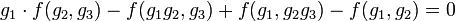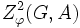# 2-cocycle for a group action

This term is defined, and makes sense, in the context of a group action on an Abelian group. In particular, it thus makes sense for a linear representation of a group

## Definition

Let$G$ be a group acting (on the left) on an abelian group$A$ via a homomorphism of groups$\varphi:G \to \operatorname{Aut}(A)$ where$\operatorname{Aut}(A)$ is the automorphism group of$A$.

### Explicit definition

A 2-cocycle for the action is a function$f:G \times G \to A$ satisfying:$\!\varphi(g_1)(f(g_2,g_3)) + f(g_1,g_2g_3) = f(g_1g_2,g_3) + f(g_1,g_2)$

If we suppress$\varphi$ and use$\cdot$ for the action, we can rewrite this as:$\!g_1 \cdot f(g_2,g_3) + f(g_1,g_2g_3) = f(g_1g_2,g_3) + f(g_1,g_2)$ or equivalently:$\! g_1 \cdot f(g_2,g_3) - f(g_1g_2,g_3) + f(g_1,g_2g_3) - f(g_1,g_2) = 0$

Note that a function$f:G \times G \to A$ (without any conditions) is sometimes termed a 2-cochain for the group action.

### Definition as part of the general definition of cocycle

A 2-cocycle for a group action is a special case of a cocycle for a group action, namely$n = 2$. This, in turn, is the notion of cocycle corresponding to the Hom complex from the bar resolution of$G$ to$A$ as$\mathbb{Z}G$-modules.

### Group structure

The set of 2-cocycles for the action of$G$ on$A$ forms a group under pointwise addition. This group is denoted$Z^2_\varphi(G,A)$ where$\varphi$ is the action. If the action is understood from the context, it can simply be denoted as$Z^2(G,A)$.

### As a group of homomorphisms

For any group$G$, we can construct a$\mathbb{Z}G$-module$K$ such that for any abelian group$A$, the group of 2-cocycles$f:G \times G \to A$ can be identified with the group of$\mathbb{Z}G$-module maps from$K$ to$A$.

## Particular cases and variations

Case or variation Condition for 2-coycle Further information$G$ acts trivially on$A$ (i.e., every element of$G$ fixes every element of$A$)$\! f(g_2,g_3) + f(g_1,g_2g_3) = f(g_1g_2,g_3) + f(g_1,g_2)$ 2-cocycle for trivial group action$G$ is abelian with additive notation for its group operation, and it acts trivially on$A$$\! f(g_2,g_3) + f(g_1,g_2 + g_3) = f(g_1 + g_2,g_3) + f(g_1,g_2)$

## Examples

### Extreme examples

• If$A$ is the trivial group, the group of 2-cocycles is the trivial group, with the only 2-cocycle being the map that sends every pair of elements of$G$ to the zero element of$A$.
• If$G$ is the trivial group, the group of 2-cocycles is isomorphic to the group$A$, with each 2-cocycle being identified with its image value in$A$.

### Other examples

Acting group$G$ Group$A$ acted upon Action Group of 2-cocycles
trivial group any group$A$ trivial action isomorphic to$A$, where a 2-cocycle is identified with its image point
any group$G$ trivial group trivial action trivial group -- the unique 2-cocycle that sends everything to the zero element
cyclic group:Z2 cyclic group:Z2 trivial action  ?

## Relation with other properties

### Stronger properties

Property Meaning Proof of implication Proof of strictness (reverse implication failure) Capture of difference Intermediate notions
2-coboundary for a group action$f(g,h) := g \cdot \alpha(h) - \alpha(gh) + \alpha(g)$ for some function$\alpha:G \to A$ 2-coboundary implies 2-cocycle 2-cocycle not implies 2-coboundary The quotient of the group of 2-cocycles by the group of 2-coboundaries is termed the second cohomology group |FULL LIST, MORE INFO
symmetric 2-cocycle for a group action$f$ is a 2-cocycle and is a symmetric function of both its inputs
normalized 2-cocycle for a group action$f$ is a 2-cocycle and if either of the inputs to$f$ is the identity element of$f$, the output of$f$ is zero

## Importance

### Extension involving an abelian normal subgroup

Let$E$ be a group with an abelian normal subgroup isomorphic to (and explicitly identified with)$A$, and a quotient isomorphic to (and explicitly identified with)$G$, such that the induced action of the quotient$G$ on$A$ (in the sense of action by conjugation, see quotient group acts on abelian normal subgroup) is as described. Let$S$ be a system of coset representatives for$G$ in$E$ with$s: G \to S$ being the representation map. Then, define$f: G \times G \to A$ such that$\! s(gh) = f(g,h)s(g)s(h)$

In other words,$f$ measures the extent to which the collection of coset representatives fails to be closed under multiplication.

Such an$f$ is a 2-cocycle.

Note that for a particular choice of$E$, all the 2-cocycles obtained by different choices of$S$ will form a single coset of the coboundary group, that is, any two such cocycles will differ by a coboundary. Thus, in particular, we can intrinsically associate, to every extension$E$ with abelian normal subgroup$A$ and quotient$G$, an element of the second cohomology group.

Further information: second cohomology group